﻿ RC & RL Time Constant Calculator ﻿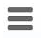# RC & RL Time Constant Calculator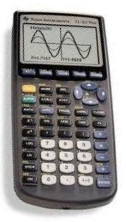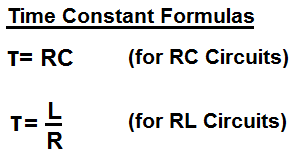### RC Time Constant Calculator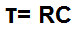pF nF µF F

0.0000000000s

### RL Time Constant Calculator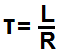pH nH µH H

0.0000000000s

The Time Constant Calculator calculates the time constant for either an RC (resistor-capacitor) circuit or an RL (resistor-inductor) circuit.

The time constant represents the amount of time it takes for a capacitor (for RC circuits) or an inductor (for RL circuits) to charge or discharge 63%.

A user enters in the resistance and either the capacitance or inductance and the time constant value is automatically computed. The resultant value is given above in unit seconds (s).

Related Resources

﻿